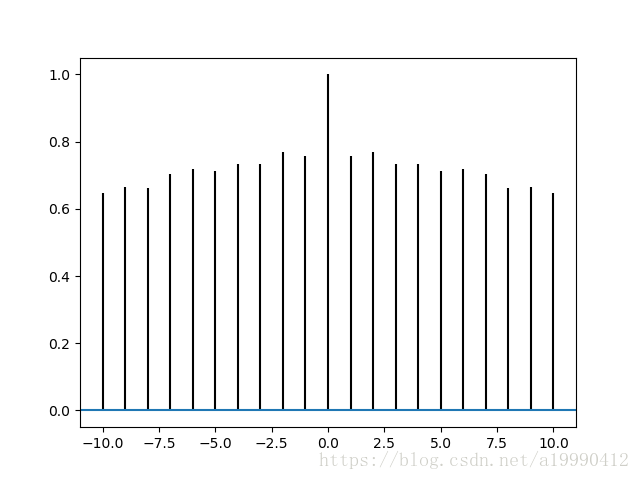# Plt.acorr() Function Error: ValueError: object too deep for desired array

sketch

Note that the input data needs to be one-dimensional. Otherwise, it’s strange to report an error（ Don’t ask me why I know)

Correct code:

``````import matplotlib.pyplot as plt
import numpy as np
data = np.random.random(100)
plt.acorr(data)
plt.show()``````Error code:

``````import matplotlib.pyplot as plt
import numpy as np
data = np.random.random((1, 100))
plt.acorr(data)
plt.show()``````